CAT  >  Maths and Logical Reasoning Test- 4

# Maths and Logical Reasoning Test- 4

Test Description

## 15 Questions MCQ Test TISS-NET Section Wise & Full Mock Test Series | Maths and Logical Reasoning Test- 4

Maths and Logical Reasoning Test- 4 for CAT 2022 is part of TISS-NET Section Wise & Full Mock Test Series preparation. The Maths and Logical Reasoning Test- 4 questions and answers have been prepared according to the CAT exam syllabus.The Maths and Logical Reasoning Test- 4 MCQs are made for CAT 2022 Exam. Find important definitions, questions, notes, meanings, examples, exercises, MCQs and online tests for Maths and Logical Reasoning Test- 4 below.
Solutions of Maths and Logical Reasoning Test- 4 questions in English are available as part of our TISS-NET Section Wise & Full Mock Test Series for CAT & Maths and Logical Reasoning Test- 4 solutions in Hindi for TISS-NET Section Wise & Full Mock Test Series course. Download more important topics, notes, lectures and mock test series for CAT Exam by signing up for free. Attempt Maths and Logical Reasoning Test- 4 | 15 questions in 15 minutes | Mock test for CAT preparation | Free important questions MCQ to study TISS-NET Section Wise & Full Mock Test Series for CAT Exam | Download free PDF with solutions
 1 Crore+ students have signed up on EduRev. Have you?
Maths and Logical Reasoning Test- 4 - Question 1

### The ratio of the three numbers is 2 : 3: 4. If the sum of its squares is 1856, then the number are:

Detailed Solution for Maths and Logical Reasoning Test- 4 - Question 1

Let the numbers be 2x,3x,4x.

Thus the sum of their square is 1856

Therefore (2x)2+(3x)2+(4x)2=1856

Or, 4x2+9x2+16x2=1856

Or, 29x2 = 1856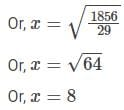Thus the numbers are 2 x 8=16, 3 x 8=24,4 x 8=32

Hence, the correct option is (B).

Maths and Logical Reasoning Test- 4 - Question 2

### The value of sin (45° + θ) – cos (45° – θ) is:

Detailed Solution for Maths and Logical Reasoning Test- 4 - Question 2

Given:

sin(45° + θ) – cos(45° – θ)

= sin{90° – (45° – θ)} – cos (45° – θ)

= cos (45° – θ) – cos (45° – θ) {∵sin(90 – A) = cos A }

= 0

Hence, the correct option is (B).

Maths and Logical Reasoning Test- 4 - Question 3

### In the following question, select the missing number from the given series.1, 2, 6, 42, ?

Detailed Solution for Maths and Logical Reasoning Test- 4 - Question 3

Here, the logic followed is:

1 × 2 = 2

2 × 3 = 6

6 × 7 = 42

42 × 43 = 1806

Hence, the correct option is (C).

Maths and Logical Reasoning Test- 4 - Question 4

Pointing to a child, a lady named Sheela said, “He is the son of my maternal grandmother’s only daughter”. How is the child’s father related to Sheela?

Detailed Solution for Maths and Logical Reasoning Test- 4 - Question 4

The possible tree diagram will be: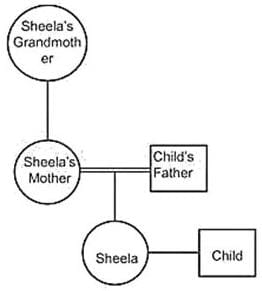So, child’s father is the ‘father’ of Sheela.

Hence, the correct option is (A).

Maths and Logical Reasoning Test- 4 - Question 5

Six friends are sitting around a circular table facing towards the center. A and B sitting opposite to each other. C sits exactly in the middle of B and D. D sits second to the right of F. D and E are sitting opposite to each other. Who among the following is sitting immediate left of A?

Detailed Solution for Maths and Logical Reasoning Test- 4 - Question 5

1) A and B sitting opposite to each other.

2) C sits exactly in the middle of B and D.

3) D sits second to the right of F.

4) D and E are sitting opposite to each other.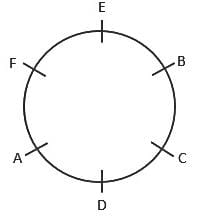So, F is sitting immediate left of A.

Hence, the correct option is (B).

Maths and Logical Reasoning Test- 4 - Question 6

Direction: In the following question, four words are given, out of which three are alike in a certain manner and the fourth one is different. Choose the odd one.

Detailed Solution for Maths and Logical Reasoning Test- 4 - Question 6

Except, Pentagon, all other shapes have four sides. Where as, pentagon has five sides.

Hence, the correct option is (D).

Maths and Logical Reasoning Test- 4 - Question 7

In the following question, select the odd letter/letters from the given alternatives.

Detailed Solution for Maths and Logical Reasoning Test- 4 - Question 7

(A) D + 4 = H; H + 5 = M

(B) G + 4 = K; K + 4 = O

(C) I + 4 = M; M + 4 = Q

(D) L + 4 = P; P + 4 = T

Hence, the correct option is (A).

Maths and Logical Reasoning Test- 4 - Question 8

Study the data carefully and answer the questions given below: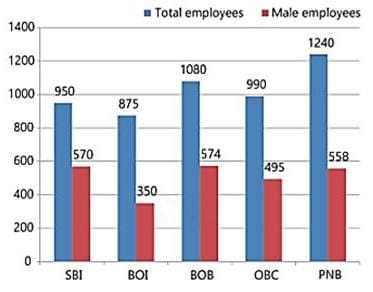The number of female employees working in OBC and PNB together is approximately how much percentage less than the total number of employees working in PNB?

Detailed Solution for Maths and Logical Reasoning Test- 4 - Question 8

The number of female employees working in OBC = 990 – 495 = 495

The number of female employees working in PNB = 1240 – 558 = 682

The number of total employees working in PNB = 1240

Percentage less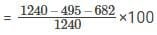= 5. 08% ≈ 5%

Hence, the correct option is (C).

Maths and Logical Reasoning Test- 4 - Question 9

Direction: Study the data carefully and answer the questions given below: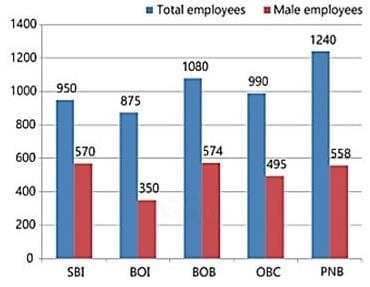What is the ratio of number of male employees who are working in SBI and BOI together to the number of female employees who are working in same bank?

Detailed Solution for Maths and Logical Reasoning Test- 4 - Question 9

Male employees working in SBI = 570

Male employees working in BOI = 350

Female employees working in SBI = 950 – 570 = 380

Female employees working in BOI = 875 – 350 = 525

Ratio = 570 + 350 : 380 + 525

= 920 : 905 = 184 : 181

Hence, the correct option is (C).

Maths and Logical Reasoning Test- 4 - Question 10

Direction: Study the data carefully and answer the questions given below: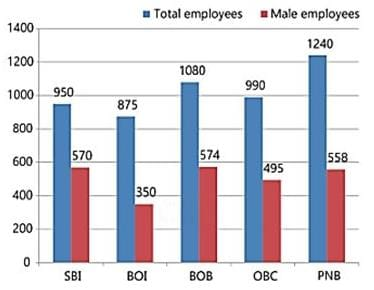What is the average number of female employees working in all the banks?

Detailed Solution for Maths and Logical Reasoning Test- 4 - Question 10

Female employees working in SBI = 950 – 570 = 380

Female employees working in BOI = 875 – 350 = 525

Female employees working in BOB = 1080 – 574 = 506

Female employees working in OBC = 990 – 495 = 495

Female employees working in PNB = 1240 – 558 = 682

Average number of employees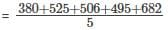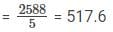Hence, the correct option is (D).

Maths and Logical Reasoning Test- 4 - Question 11

Direction: Study the data carefully and answer the questions given below: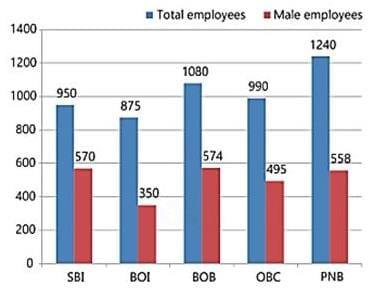The total number of employees who are working in BOI is 80% of the total number of employees who are working in BOB. Find the percentage of female employees who are working in BOI if the number of male employees is 648.

Detailed Solution for Maths and Logical Reasoning Test- 4 - Question 11

Total number of employees who are working in BOB = 1080

Total number of employees who are working in BOI = 1080 × 80% = 864

Percentage of male employees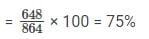Percentage of female employees = 100 – 75% = 25%

Hence, the correct option is (D).

Maths and Logical Reasoning Test- 4 - Question 12

Find the wrong term in following series.

256, 289, 324, 351, 400, 441.

Detailed Solution for Maths and Logical Reasoning Test- 4 - Question 12

The logic follows here is:-

162 = 256

172 = 289

182 = 324

192 = 361

202 = 400

212 = 441

Thus, Clearly 351 is the wrong number in given series as it must be 361.

Hence, the correct option is (A).

Maths and Logical Reasoning Test- 4 - Question 13

What should come in the place of question mark (?) in the following number series?

27, 40.5, 60.75, 91.125, ?,

Detailed Solution for Maths and Logical Reasoning Test- 4 - Question 13

Pattern is as follows,

27 × 1.5 = 40.5

40.5 × 1.5 = 60.75

60.75 × 1.5 = 91.125

91.125 × 1.5 = 136.6875

Hence, the correct option is (B).

Maths and Logical Reasoning Test- 4 - Question 14

In the following question, four words are given, out of which three are alike in a certain manner and the fourth one is different. Choose the different one.

Detailed Solution for Maths and Logical Reasoning Test- 4 - Question 14

All plants except cactus, are aquatic plants. Whereas, cactus is found in the desert.

Hence, the correct option is (B).

Maths and Logical Reasoning Test- 4 - Question 15

Ankit walks 7 km from school, turns right, and walks 5 km, then he turns left and walks 11 km. Again turns right and walks for 5 km. In the end, he notices that he is facing towards the east. In which direction was the school from the present place?

Detailed Solution for Maths and Logical Reasoning Test- 4 - Question 15

Drawing the diagram according to the given information,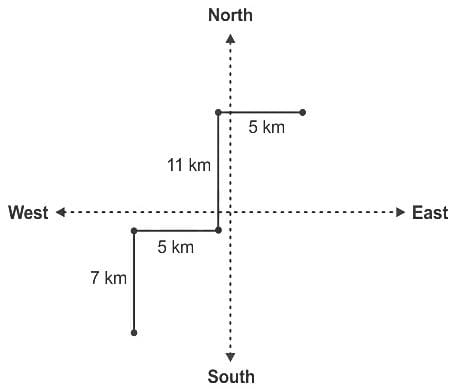So, the school is in the southwest direction to the present place.

Hence, the correct option is (B).

## TISS-NET Section Wise & Full Mock Test Series

28 tests
 Use Code STAYHOME200 and get INR 200 additional OFF Use Coupon Code
Information about Maths and Logical Reasoning Test- 4 Page
In this test you can find the Exam questions for Maths and Logical Reasoning Test- 4 solved & explained in the simplest way possible. Besides giving Questions and answers for Maths and Logical Reasoning Test- 4, EduRev gives you an ample number of Online tests for practice

## TISS-NET Section Wise & Full Mock Test Series

28 tests

### How to Prepare for CAT

Read our guide to prepare for CAT which is created by Toppers & the best Teachers